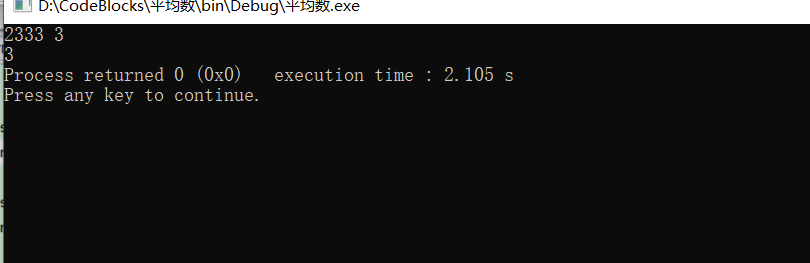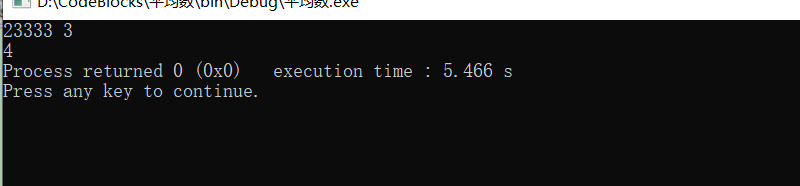2021-06-29 19:47

# ，这一题真的不会，，，，，，，，

请分别用
int cnum(char *p, char c); 和 void cnum(char *p, char c, int *n);
两种方法函数原型分别来编写该程序。

• 点赞
• 写回答
• 关注问题
• 收藏
• 邀请回答

#### 2条回答默认 最新

•已采纳
``````#include<stdio.h>
int cnum(char * p,char c)
{
int i=0;
int count=0;
do
{
if(p[i]==c)
{
count++;
}
}while(p[++i]!='\0');
return count;
}
int main()
{
int i,a,b,sum=0;
char s,c;
scanf("%s %c",s,&c);
printf("%d",cnum(s,c));
return 0;
}

``````第二种：

``````#include<stdio.h>
void cnum(char * p,char c, int *n)
{
int i=0;
do
{
if(p[i]==c)
{
(*n)++;
}
}
while(p[++i]!='\0');

}
int main()
{
int i,a,b,sum=0;
char s,c;
scanf("%s %c",s,&c);
cnum(s,c,&sum);
printf("%d",sum);
return 0;
}

``````点赞 打赏 评论
•``````#include<stdio.h>
int cnum(char * p,char c)
{
int count = 0;
for(int i=0;p[i] != 0;i++)
if(p[i] == c)
count++;
return count;
}

void cnum(char * p,char c,int *n)
{
*n = 0;
for(int i=0;p[i] != 0;i++)
if(p[i] == c)
*n++;
}

int main()
{
char a;
char c;
printf("请输入字符串:");
scanf("%s",a);
getchar();
printf("请输入搜索字符:");
scanf("%c",&c);
int count = cnum(a,c);
printf("%c字符共出现%d次。\n",c,count);
count = 0;
cnum(a,c,&count);
printf("%c字符共出现%d次。\n",c,count);
return 0;
}

``````
点赞 打赏 评论